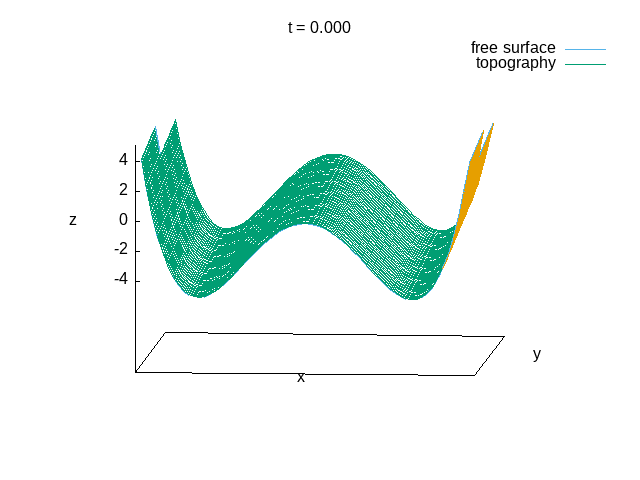# Flow rates for multiple rivers

In this example, we impose different flow rates on different rivers situated on the same boundary of a Saint-Venant simulation.

#include "saint-venant.h"
#include "discharge.h"

The domain is 10 metres squared, centered on the origin. Time is in seconds.

#define LEVEL 7

int main()
{
size (10.);
origin (- L0/2., - L0/2.);
G = 9.81;
N = 1 << LEVEL;
run();
}

## Initial conditions

We chose a reasonably complicated river bed with two “valleys” (see Figure below).

We allocate a new field river which is set to different values (1 and 2) for each of the rivers.

scalar river[];

event init (i = 0)
{

We start with a dry riverbed, so that the problem does not have a natural timescale the Saint-Venant solver can use. We set a maximum timestep to set this timescale.

  DT = 1e-2;

foreach() {
zb[] = 0.05*pow(x,4) - x*x + 2. + 0.2*(y + Y0);
river[] = x < 0 ? 1 : 2;
}
}

## Boundary conditions

We impose inflow/outflow on both the top and bottom boundary. In addition, the tangential velocity on the top boundary u.t is set to zero.

u.n[top] = neumann(0);
u.t[top] = dirichlet(0);

u.n[bottom] = neumann(0);

To impose a given flow rate for the two rivers on the top boundary, we compute the elevation \eta of the water surface necessary to match this flow rate. This gives two elevation values eta1 and eta2, one for each river. The flow rates are set to 4 and 2 m3/sec for river 1 and 2 respectively.

double eta1, eta2;

event inflow (i++) {
eta1 = eta_b (4, top, river, 1);
eta2 = eta_b (2, top, river, 2);

Once we have the required values for the water surface elevations at the top boundary of both riverbeds, we impose them on both h and \eta=h+z_b.

  h[top] = max ((river[] == 1. ? eta1 : eta2) - zb[], 0.);
eta[top] = max ((river[] == 1. ? eta1 : eta2) - zb[], 0.) + zb[];
}

## Outputs

We compute the evolution of the water volumes in both riverbeds.

event volume (i += 10) {
double volume1 = 0, volume2 = 0;
foreach(reduction(+:volume1) reduction(+:volume2)) {
double dv = h[]*sq(Delta);
if (x < 0) volume1 += dv;
else volume2 += dv;
}
fprintf (stderr, "%g %g %g %g %g\n",
t, volume1, volume2, eta1, eta2);
}

We use gnuplot to produce an animation of the water surface.

event init_animation (i = 0) {
printf ("set view 80,05\n"
"set xlabel 'x'\n"
"set ylabel 'y'\n"
"set zlabel 'z'\n"
"set hidden3d; unset ytics ; unset xtics\n");
}

event animation (t <= 1; i += 10) {
double dx = 2.*L0/N, dy = dx;
printf ("set title 't = %.3f'\n"
"sp [%g:%g][%g:%g][-5:5] '-'"
" u 1:2:($3+$4-.05) t 'free surface' w l lt 3,"
" '' u 1:2:4 t 'topography' w l lt 2\n",
t, X0, -X0, Y0, -Y0);
for (double x = X0;  x <= X0 + L0; x += dx) {
for (double y = Y0; y <= Y0 + L0; y += dy)
printf ("%g %g %g %g\n",
x, y, interpolate (h, x, y),  interpolate (zb, x, y));
putchar ('\n');
}
printf ("e\n"
"pause %.5lf \n\n", 0.);
}

## Results

set key top left
set xlabel 'Time'
set ylabel 'Volume'
f(x)=a*x+b
fit [0.2:] f(x) 'log' u 1:2 via a,b
g(x)=c*x+d
fit [0.2:] g(x) 'log' u 1:3 via c,d
title1=sprintf("f(x) = %1.3f*t + %1.3f", a, b)
title2=sprintf("g(x) = %1.3f*t + %1.3f", c, d)
plot 'log' u 1:2 t 'river 1', f(x) t title1, \
'log' u 1:3 t 'river 2', g(x) t title2Evolution of water volumes in both rivers (script)

reset
set term gif animate
set output 'movie.gif'
load './out'Animation of the free surface. (script)